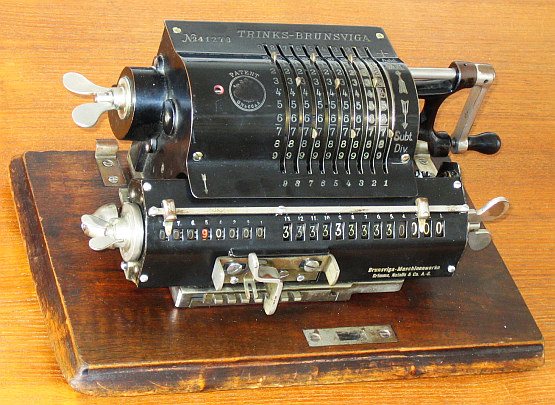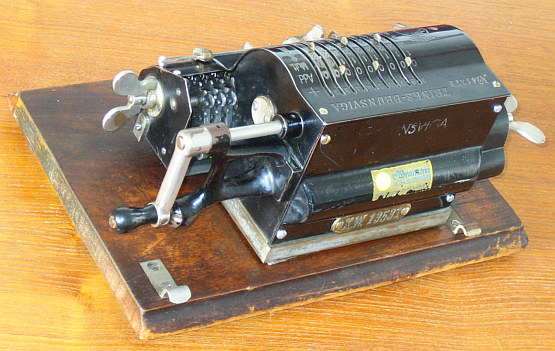previous <<==>> next

BRUNSVIGA-B
» TRINKS-BRUNSVIGA «The (? Sewing-Machine ?) BOARD is cut on its right side !Have a look INSIDE ...
 TECHNICAL DATA of the Mechanical Calculator BRUNSVIGA-B ************************************************************************** » TRINKS-BRUNSVIGA « Serial Number: 41270 ( Chassis = 847 ) Dimensions: (ca.) Width = 13_3/4" / 35 cm ( with ) Depth = 8_1/2" / 22 cm ( its ) Height = 6 " / 14 cm ( BOARD ) Weight: (ca.) 21 lbs / 9.5 g ( with its wooden BOARD ) Mechanics: Pin-Wheel / Sprossenrad 10s Carry Mechanism Counter Stepping Mechanism + Animation Functions: Add, Subtract, Multiply, Divide Registers: Input = 9 Decimals Counter = 8 Decimals (NO Carry; Neg.Figures RED) Arithmetic = 13 Decimals Manufacturer: BRUNSVIGA MASCHINENWERKE Grimme, Natalis & Co. AG. Braunschweig Germany 1910 H O W T O U S E the BRUNSVIGA-B ... ******************************************* BASIC SETTINGS: =============== (a) Crank: ---------- For addition (+) the crank is turned clockwise; for subtraction (-) the crank is turned counter-clockwise. In the idle position the crank is down & locked. To make one or more turns with the crank, pull out the handle to unlock. When finished the turn(s), let the handle snap into lock again. The locked down position only makes other functions accessible. REMARK: Every started turn has to be finished completely! Accidentally started turns are correctible somehow. (b) Clearing Registers: ----------------------- The input register is manipulated by 10 levers. The input can be cleared with the wing-nut on the left side of the body. The arithmetic register is to clear with the wing-nut on the right side of the carriage. The counter is to clear with the wing-nut on the left side of the carriage. (c) Shifting the Carriage: -------------------------- With the 2-grip lever is movable one step right or one step left. The left position "1" is the "Start Position" of the carriage. (d) Counting Direction: ----------------------- It depends of the 1st turn of the crank what happens: When (for addition) the crank's 1st turn is clockwise, the counter display shows white figures; when (for subtraction) the crank's 1st turn is counter-clockwise, the counter display shows red figures. The counter has NO 10s-Carry! Under the red window it shows a (+) or (-) ADDITION & SUBTRACTION: ======================= Example: 123 + 45 - 6 = 162 Clear input, counter and arithmetic units. Carriage in position 1. ADD: Enter the first number (123) in the far right of the input unit. Make a positive (clockwise) turn with the crank to transfer the number into arithmetic unit. The counting unit displays the figure 1. Enter the second number (45). Make a positive (clockwise) turn with the crank to add the number. The arithmetic unit displays the intermediate sum (168) and the counting unit displays the figure 2. SUBTRACT: Enter the third number (6). Make a negative (counter-clockwise) turn with the crank. The arithmetic unit displays the result (162) and the counting unit is decreased by 1. REMARK: NEGATIVE RESULTS are displayed in the arithmetic unit as the COMPLEMENT of the next higher 10, 100, 1000, ... Example: -12 = 99...9988 MULTIPLICATION: =============== Example: 123 x 45 = 5535 Clear input, counter and arithmetic units. Enter the multiplicand (123) in the far right of the input unit. The multiplicator (45) has two digits, so the carriage is shifted to position 2 by pressing the 2-grip lever forward. Make positive (clockwise) turns with the crank, until the first figure of the multi- plicator (4) will appear in the 2nd position of the counter unit. Shift the carriage to position 1 by pressing the 2-grip lever backward. Repeat making positive turns with the crank, until the second figure of the multiplicator (5) appears in the 1st position of the counter unit. The multiplication is done: The multiplicand (123) stays in the input unit, the multiplicator (45) in the counter and the result (5535) is in the arithmetic unit. DIVISION: ========= Example: 22 : 7 = 3.1428571 Remainder 3 Division requires 3 steps: (1st) To Set the Dividend into Arithmetic Unit: ----------------------------------------------- For the maximum number of decimals, pull out the carriage to the far right position. Enter the dividend (22) in the far right of the input unit. Make a positive (clockwise) turn with the crank to transfer into arithmetic unit. (2nd) To Set the Divisor into Input Unit: ----------------------------------------- Clear counter & input units. Enter the divisor (7) above the dividend (22). (3rd) To Divide: ---------------- Make negative (counter-clockwise) turns (the counter will now show red figures!) with the crank until the arithmetic unit shows an "underflow". Make one positive (clockwise) turn with the crank. Move the carriage to the next left position. Repeat this procedure until the required number of decimals are calculated... The result (3.1428571) is in the counter unit ( in RED ), and the remainder (3) is in the arithmetic unit. The divisor (7) stays in the input unit, therefore an additional decimal can be estimated ... ( 4, cause 4 x 7 = 28). impressum: ************************************************************************** © C.HAMANN http://public.BHT-Berlin.de/hamann 04/14/14